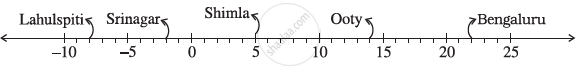Share

# Following Number Line Shows the Temperature in Degree Celsius (°C) at Different Places on a Particular Day Observe this Number Line and Write the Temperature of the Places Marked on It. - CBSE Class 7 - Mathematics

ConceptIntroduction of Integer

#### Question

Following number line shows the temperature in degree celsius (°C) at different places on a particular dayObserve this number line and write the temperature of the places marked on it.

#### Solution

1) By observing the given data, the temperatures of these cities are as follows.

Lahulspiti  : −8°C

Srinagar   : −2°C

Shimla     :  5°C

Ooty       :  14°C

Bangalore : 22°C

Is there an error in this question or solution?

#### APPEARS IN

NCERT Solution for Mathematics for Class 7 (2018 to Current)
Chapter 1: Integers
Ex. 1.10 | Q: 1.1 | Page no. 4
Solution Following Number Line Shows the Temperature in Degree Celsius (°C) at Different Places on a Particular Day Observe this Number Line and Write the Temperature of the Places Marked on It. Concept: Introduction of Integer.
S